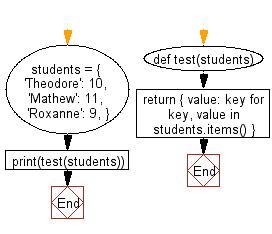﻿ Python: Invert a dictionary with unique hashable values - w3resource# Python: Invert a dictionary with unique hashable values

## Python dictionary: Exercise-72 with Solution

Write a Python program to invert a dictionary with unique hashable values.

• Use dictionary.items() in combination with a list comprehension to create a new dictionary with the values and keys inverted.

Sample Solution:

Python Code:

``````def test(students):
return { value: key for key, value in students.items() }

students = {
'Theodore': 10,
'Mathew': 11,
'Roxanne': 9,
}
print(test(students))
```
```

Sample Output:

```{10: 'Theodore', 11: 'Mathew', 9: 'Roxanne'}
```

Flowchart:## Visualize Python code execution:

The following tool visualize what the computer is doing step-by-step as it executes the said program:

Python Code Editor:

Have another way to solve this solution? Contribute your code (and comments) through Disqus.

What is the difficulty level of this exercise?

Test your Programming skills with w3resource's quiz.

﻿

## Python: Tips of the Day

Decapitalizes the first letter of a string:

Example:

```def tips_decapitalize(s, upper_rest=False):
return s[:1].lower() + (s[1:].upper() if upper_rest else s[1:])
print(tips_decapitalize('PythonTips'))
print(tips_decapitalize('PythonTips', True))
```

Output:

```pythonTips
pYTHONTIPS
```